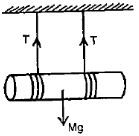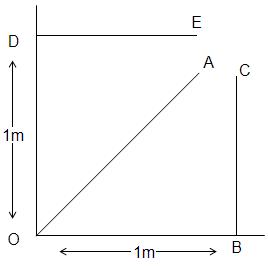Two balls of equal masses have a head-on collision with speed 6 m/s each. If the coefficient of restitution is 1/3, the speed of each ball after impact will be

1. 18 m/s

2. 2 m/s

3. 6 m/s

4. data insufficient

Concept Questions :-

Linear momentum
High Yielding Test Series + Question Bank - NEET 2020

Difficulty Level:

A wheel is rotating 900 rpm about its axis. When power is cut off it comes to rest in 1 min. The angular retardation in rad/s2 is

1. $\mathrm{\pi }/2$

2. $\mathrm{\pi }/4$

3. $\mathrm{\pi }/6$

4. $\mathrm{\pi }/8$

Concept Questions :-

Rotation motion : Introduction
High Yielding Test Series + Question Bank - NEET 2020

Difficulty Level:

A particle of mass 2 kg located at the position  m has a velocity of 2 m/s. Its angular momentum about z-axis in kg-${\mathrm{m}}^{2}/\mathrm{s}$ is :

1. +4

2. +8

3. -4

4. -8

Concept Questions :-

Angular momentum
High Yielding Test Series + Question Bank - NEET 2020

Difficulty Level:

A cylinder of mass 'M' is suspended by two strings wrapped around it as shown. The acceleration 'a' and the tension T when the cylinder falls and the string unwinds itself is

1.

2.

3.

4.Concept Questions :-

Torque
High Yielding Test Series + Question Bank - NEET 2020

Difficulty Level:

An automobile engine develops 100 kW when rotating at a speed of 1800 rev/min. What torque does it deliver ?

1. 350 N-m

2. 440 N-m

3. 531 N-m

4. 628 N-m

Concept Questions :-

Torque
High Yielding Test Series + Question Bank - NEET 2020

Difficulty Level:

In an orbital motion, the angular momentum vector is

2. Parallel to the linear momentum

3. In the orbital plane

4. Perpendicular to the orbital plane

Concept Questions :-

Angular momentum
High Yielding Test Series + Question Bank - NEET 2020

Difficulty Level:

A particle of mass m moves with a constant velocity along 3 different paths DE, OA & BC. Which of the following statements is not correct about its angular momentum about point O?(A) It is zero when it is at A and moving along OA.

(B) The same at all points along the line DE.

(C) Of the same magnitude but oppositely directed at B and  D.

(D) Increases as it moves along the line BC.

Concept Questions :-

Angular momentum
High Yielding Test Series + Question Bank - NEET 2020

Difficulty Level:

High Yielding Test Series + Question Bank - NEET 2020

Difficulty Level:

A sphere is rolling down a plane of inclination $\theta$ to the horizontal.  The acceleration of its centre down the plane is

(1) g sin $\theta$

(2) less than g sin $\theta$

(3) greater than g sin $\theta$

(4) zero

High Yielding Test Series + Question Bank - NEET 2020

Difficulty Level:

A body at rest breaks into two pieces of equal masses.  The parts will move

(1) in same direction

(2) along different lines

(3) in opposite directions with equal speeds

(4) in opposite directions with unequal speeds